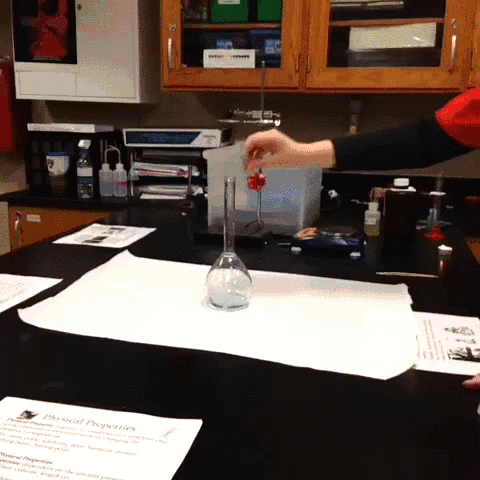## Chemical Reaction Quiz

Science Quiz by www.ChemistryNotesInfo.comSource www.reddit.com
This Chemical Reaction Quiz part16 is based on chemical reactions.

#### 1. Chemical reaction always gives-

Reactants
A change of state
A different substance
Water
All of the above

#### 2. From given below, 4-balanced chemical equations, which is example of combustion reaction-

2Mg + O2 → 2MgO
2N2 + 3H2 → 2NH3
CaO + H2O → Ca(OH)2
2Na + Cl2 → 2NaCl
2CH4 + 4O2 → 2CO2 + 4H2O

#### 3. To balance a chemical equation, you should need to change-

Chemical Formulas
Direction of the arrow
Coefficients
Subscripts
Superscripts

#### 4. In chemistry, if you try to balance a chemical equation by changing its subscripts, than you change the-

Atomic number
Atomic Mass
Mass of the reactants
Number of molecules of product
Identity of the compound

#### 5. From given below, 4-balanced chemical equations, which is example of double displacement reaction-

NH4Cl → NH3 + HCl
Cd(NO3)2 + Na2S → CdS + 2NaNO3
2Mg + O2 → 2MgO
2H2O2 → 2H2O + O2
2N2 + 3H2 → 2NH3

#### 6. Chemical Reaction, KClO3 → KCl + O2 is a-

Synthesis Reaction
Decomposition Reaction
Combustion Reaction
Single Displacement Reaction
Double Displacement Reaction

Lavoisier
Galileo
Newton
Archimedes
Pasteur

#### 8. Combination reactions (also known as Synthesis Reaction) always-

Involve an element and an ionic compound
Require oxygen gas
Use only one reactant
Form only one product
form only two product

#### 9. In a chemical reaction, the arrow means-

A reaction is happening
The equation is balanced
The products flow into reactants
The two sides are equal
All of the above

#### 10. In a decomposition reaction-

Energy in the form of light or heat is often released
Reactants are commonly two ionic compounds in the aqueous solution
one of the reactant is generally water
Form only one product
Reactants in the chemical reaction are usually a metal and a nonmetal